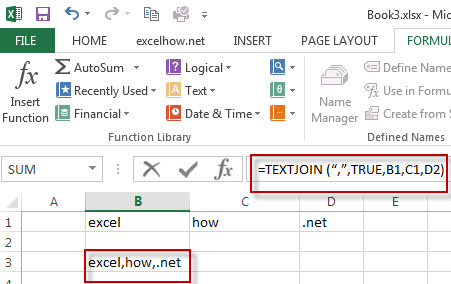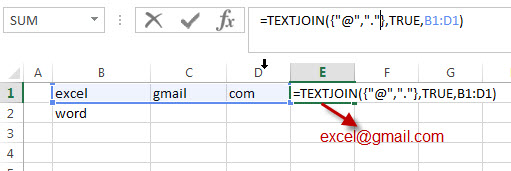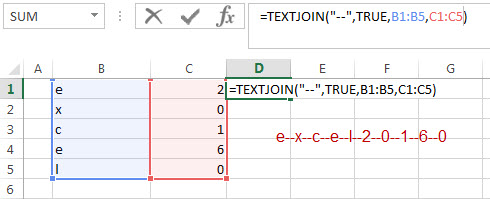# Excel TextJoin Function

This post will guide you how to use Excel TEXTJOIN function with syntax and examples in Microsoft excel. how to concatenate strings using the TEXTJOIN function in excel 2016. we can use CONCATENATE function to join text in differenct cells in 2013 or lower version excel.

## Description

The Excel TEXTJOIN function joins two or more text strings together and separated by a delimiter. you can select an entire range of cell references to be combined in excel 2016. and you can also specify a en empty string as delimiter to include between each texts in different cells.

The TEXTJOIN function is a build-in function in Microsoft Excel and it is categorized as a Text Function.

The TEXTJOIN function is only available in Excel 2016.

## Syntax

The syntax of the TEXTJOIN function is as below:

`= TEXTJOIN  (delimiter, ignore_empty,text1,[text2])`

Where the TEXTJOIN function arguments are:
delimiter -This is a required argument. It can be a text string or empty string to join text values with a given delimiter, it can be a space,comma,hash or other text string.
Ignore_empty – This is a required argument.  If TRUE, empty cells or string value should be ignored.
Text1/Text2– This is a required argument.  One or more strings that you want to join together.
Note: the text arguments should not exceed 252 and the length of resulting string should not exceed the cell limit of 32767.

## Excel TEXTJOIN Function Examples

The below examples will show you how to use Excel TEXTJOIN Text function to concatenate the items of one or more text strings using a specified delimiter.

#1 To join strings in B1,C1,D1 cells, just using formula:= TEXTJOIN (“,”,TRUE,B1,C1,D2).#2 while you want to concatenate the strings by specifying multiple delimiter and order, you can use the following formula:

=TEXTJOIN({“@”,”.”,”.”},TRUE,A1:C1)#3 To join values from the multiple cell ranges with a double dash character as delimiter. using the following formula:

=TEXTJOIN(“–“,TRUE,A1:A4,B1:B4)#4 to join string in range cell A1:C1 by a comma character as delimiter and empty cells should not be ignored. using the following formula:

=TEXTJOIN(“,”,FALSE,A1:C1)

## More TEXTJOIN Formula Examples

• Remove Numeric Characters from a Cell
If you want to remove numeric characters from alphanumeric string, you can use the following complex array formula using a combination of the TEXTJOIN function, the MID function, the Row function, and the INDIRECT function..…
• remove non numeric characters from a cell
If you want to remove non numeric characters from a text cell in excel, you can use the array formula:{=TEXTJOIN(“”,TRUE,IFERROR(MID(B1,ROW(INDIRECT(“1:”&LEN(B1))),1)+0,””))}…
• How to combine Text from Two or More Cells into One Cell
If you want to combine text from multiple cells into one cell and you can use the Ampersand (&) symbol.If you are using the excel 2016, then you can use a new function TEXTJOIN function to combine text from multiple cells…

### Related Functions

• Excel TEXTJOIN function
The Excel TEXTJOIN function joins two or more text strings together and separated by a delimiter. you can select an entire range of cell references to be combined in excel 2016.The syntax of the TEXTJOIN function is as below:= TEXTJOIN  (delimiter, ignore_empty,text1,[text2])…
• Excel MID function
The Excel MID function returns a substring from a text string at the position that you specify.The syntax of the MID function is as below:= MID (text, start_num, num_chars)…
• Excel LEN function
The Excel LEN function returns the length of a text string (the number of characters in a text string).The LEN function is a build-in function in Microsoft Excel and it is categorized as a Text Function.The syntax of the LEN function is as below:= LEN(text)…
• Excel IFERROR function
The Excel IFERROR function returns an alternate value you specify if a formula results in an error, or returns the result of the formula.The syntax of the IFERROR function is as below:= IFERROR (value, value_if_error)….
• Excel ROW function
The Excel ROW function returns the row number of a cell reference.The ROW function is a build-in function in Microsoft Excel and it is categorized as a Lookup and Reference Function.The syntax of the ROW function is as below:= ROW ([reference])….
• Excel INDIRECT  function
The Excel ROW function returns the row number of a cell reference.The ROW function is a build-in function in Microsoft Excel and it is categorized as a Lookup and Reference Function.The syntax of the ROW function is as below:= ROW ([reference])….